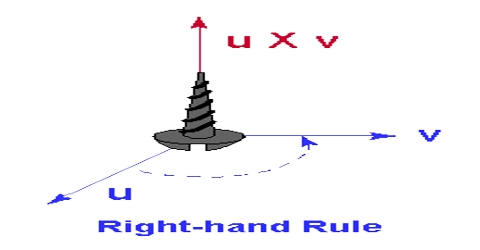Physics

# Right Handed Screw RuleRight handed screw rule: By keeping a right handed screw on the plane that contains the two vectors and if the first vector is rotated towards the second vector along the smallest angle, then the direction through which the screw rotates will be the direction of R, that means, the direction of η.

According to the above rule the direction of P x Q will be upward [Fig. 1] and that of Q  x P will be downward [Fig. 2]. That means, in the first case the director of the right handed screw will be anticlockwise and in the second case it is clockwise. Anticlockwise direction is considered as positive, whereas clockwise direction is considered as negative.Some Common rules:

(a) If α = 0°, then R = P x Q = η sin 00, in this case the two vectors will be mutually parallel to each other.

(b) If α = 90°, then R = P x Q = η PQ sin 900 = PQ, in this case two vectors will be mutually perpendicular to each other.

(c) If α = 1800, then R = P x Q = η PQ sin 1800 = 0. In this case, two vectors will be parallel and opposite to each other.